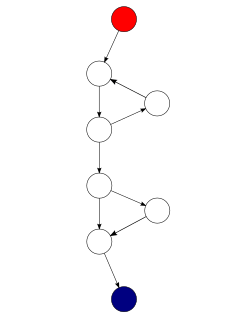# Cyclomatic complexity facts for kids

Kids Encyclopedia FactsA control flow graph of a simple program. The program begins executing at the red node, then enters a loop (group of three nodes immediately below the red node). On exiting the loop, there is a conditional statement (group below the loop), and finally the program exits at the blue node. This graph has 9 edges, 8 nodes, and 1 connected component, so the cyclomatic complexity of the program is 9 - 8 + (2*1) = 3.

Cyclomatic complexity is a measurement to see how difficult a computer program is to understand. The measurement was developed by Thomas J. McCabe, Sr. in 1976. It looks at the program's source code and measures the number of independent paths through the source code. The concept is similar to the general text complexity, which is measured by the Flesch-Kincaid Readability Test.

Cyclomatic complexity is computed using the control flow graph of the program: the nodes of the graph correspond to indivisible groups of commands of a program, and a directed edge connects two nodes if the second command might be executed immediately after the first command. Cyclomatic complexity may also be applied to individual functions, modules, methods or classes within a program.

One testing strategy, called Basis path testing by McCabe who first proposed it, is to test each linearly independent path through the program; in this case, the number of test cases will equal the cyclomatic complexity of the program.Cyclomatic complexity Facts for Kids. Kiddle Encyclopedia.